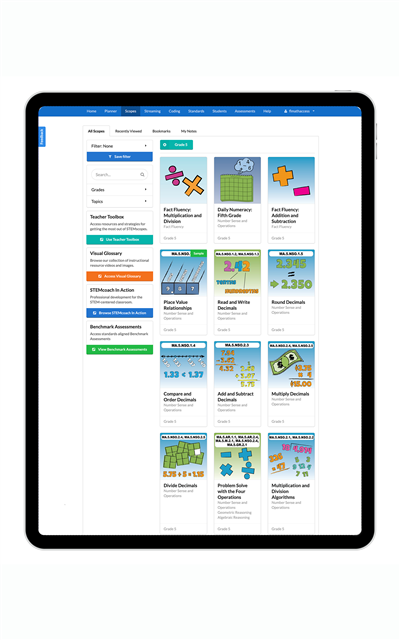This document was generated on CPALMS - www.cpalms.org
You are not viewing the current course, please click the current year’s tab.

#### Course Standards

Name Description
MA.5.AR.1.1: Solve multi-step real-world problems involving any combination of the four operations with whole numbers, including problems in which remainders must be interpreted within the context.
 Clarifications:Clarification 1: Depending on the context, the solution of a division problem with a remainder may be the whole number part of the quotient, the whole number part of the quotient with the remainder, the whole number part of the quotient plus 1, or the remainder.
MA.5.AR.1.2: Solve real-world problems involving the addition, subtraction or multiplication of fractions, including mixed numbers and fractions greater than 1.
 Clarifications:Clarification 1: Instruction includes the use of visual models and equations to represent the problem.

 Examples:Shanice had a sleepover and her mom is making French toast in the morning. If her mom had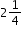loaves of bread and used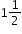loaves for the French toast, how much bread does she have left?
MA.5.AR.1.3: Solve real-world problems involving division of a unit fraction by a whole number and a whole number by a unit fraction.
 Clarifications:Clarification 1: Instruction includes the use of visual models and equations to represent the problem.

 Examples:Example: A property has a total of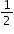acre and needs to be divided equally among 3 sisters. Each sister will receiveof an acre. Example: Kiki has 10 candy bars and plans to give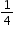of a candy bar to her classmates at school. How many classmates will receive a piece of a candy bar?
MA.5.AR.2.1: Translate written real-world and mathematical descriptions into numerical expressions and numerical expressions into written mathematical descriptions.
 Clarifications:Clarification 1: Expressions are limited to any combination of the arithmetic operations, including parentheses, with whole numbers, decimals and fractions. Clarification 2: Within this benchmark, the expectation is not to include exponents or nested grouping symbols.

 Examples:The expression 4.5 + (3×2) in word form is four and five tenths plus the quantity 3 times 2.
MA.5.AR.2.2: Evaluate multi-step numerical expressions using order of operations.
 Clarifications:Clarification 1: Multi-step expressions are limited to any combination of arithmetic operations, including parentheses, with whole numbers, decimals and fractions. Clarification 2: Within this benchmark, the expectation is not to include exponents or nested grouping symbols. Clarification 3: Decimals are limited to hundredths. Expressions cannot include division of a fraction by a fraction.

 Examples:Patti says the expression 12÷2×3 is equivalent to 18 because she works each operation from left to right. Gladys says the expression 12÷2×3 is equivalent to 2 because first multiplies 2×3 then divides 6 into 12. David says that Patti is correctly using order of operations and suggests that if parentheses were added, it would give more clarity.
MA.5.AR.2.3: Determine and explain whether an equation involving any of the four operations is true or false.
 Clarifications:Clarification 1: Problem types include equations that include parenthesis but not nested parentheses.Clarification 2: Instruction focuses on the connection between properties of equality and order of operations.

 Examples:The equation 2.5+(6×2)=16-1.5 can be determined to be true because the expression on both sides of the equal sign are equivalent to 14.5.
MA.5.AR.2.4: Given a mathematical or real-world context, write an equation involving any of the four operations to determine the unknown whole number with the unknown in any position.
 Clarifications:Clarification 1: Instruction extends the development of algebraic thinking where the unknown letter is recognized as a variable. Clarification 2: Problems include the unknown and different operations on either side of the equal sign

 Examples:The equation 250-(5×s)=15 can be used to represent that 5 sheets of paper are given to s students from a pack of paper containing 250 sheets with 15 sheets left over.
MA.5.AR.3.1: Given a numerical pattern, identify and write a rule that can describe the pattern as an expression.
 Clarifications:Clarification 1: Rules are limited to one or two operations using whole numbers.

 Examples:The given pattern 6,8,10,12… can be describe using the expression 4+2x, where x=1,2,3,4… ; the expression 6+2x, where x=0,1,2,3… or the expression 2x, where x=3,4,5,6….
MA.5.AR.3.2: Given a rule for a numerical pattern, use a two-column table to record the inputs and outputs.
 Clarifications:Clarification 1: Instruction builds a foundation for proportional and linear relationships in later grades.Clarification 2: Rules are limited to one or two operations using whole numbers.

Examples:

The expression 6+2x, where x represents any whole number, can be represented in a two-column table as shown below.

 Input (X) 0 1 2 3 Output 6 8 10 12

MA.5.DP.1.1: Collect and represent numerical data, including fractional and decimal values, using tables, line graphs or line plots.
 Clarifications:Clarification 1: Within this benchmark, the expectation is for an estimation of fractional and decimal heights on line graphs. Clarification 2: Decimal values are limited to hundredths. Denominators are limited to 1, 2, 3 and 4. Fractions can be greater than one.

 Examples:Gloria is keeping track of her money every week. She starts with \$10.00, after one week she has \$7.50, after two weeks she has \$12.00 and after three weeks she has \$6.25. Represent the amount of money she has using a line graph.
MA.5.DP.1.2: Interpret numerical data, with whole-number values, represented with tables or line plots by determining the mean, mode, median or range.
 Clarifications:Clarification 1: Instruction includes interpreting the mean in real-world problems as a leveling out, a balance point or an equal share.

 Examples:Rain was collected and measured daily to the nearest inch for the past week. The recorded amounts are 1,0,3,1,0,0 and 1. The range is 3 inches, the modes are 0 and 1 inches and the mean value can be determined as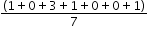which is equivalent to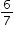of an inch. This mean would be the same if it rainedof an inch each day.
MA.5.FR.1.1: Given a mathematical or real-world problem, represent the division of two whole numbers as a fraction.
 Clarifications:Clarification 1: Instruction includes making a connection between fractions and division by understanding that fractions can also represent division of a numerator by a denominator. Clarification 2: Within this benchmark, the expectation is not to simplify or use lowest terms. Clarification 3: Fractions can include fractions greater than one.

 Examples:At Shawn’s birthday party, a two-gallon container of lemonade is shared equally among 20 friends. Each friend will have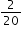of a gallon of lemonade which is equivalent to one-tenth of a gallon which is a little more than 12 ounces.
MA.5.FR.2.1: Add and subtract fractions with unlike denominators, including mixed numbers and fractions greater than 1, with procedural reliability.
 Clarifications:Clarification 1: Instruction includes the use of estimation, manipulatives, drawings or the properties of operations. Clarification 2: Instruction builds on the understanding from previous grades of factors up to 12 and their multiples.

 Examples:The sum of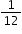and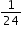can be determined as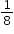,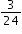,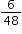or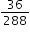by using different common denominators or equivalent fractions.
MA.5.FR.2.2: Extend previous understanding of multiplication to multiply a fraction by a fraction, including mixed numbers and fractions greater than 1, with procedural reliability.
 Clarifications:Clarification 1: Instruction includes the use of manipulatives, drawings or the properties of operations.Clarification 2: Denominators limited to whole numbers up to 20.
MA.5.FR.2.3: When multiplying a given number by a fraction less than 1 or a fraction greater than 1, predict and explain the relative size of the product to the given number without calculating.
 Clarifications:Clarification 1: Instruction focuses on the connection to decimals, estimation and assessing the reasonableness of an answer.
MA.5.FR.2.4: Extend previous understanding of division to explore the division of a unit fraction by a whole number and a whole number by a unit fraction.
 Clarifications:Clarification 1: Instruction includes the use of manipulatives, drawings or the properties of operations.Clarification 2: Refer to Situations Involving Operations with Numbers (Appendix A).
MA.5.GR.1.1: Classify triangles or quadrilaterals into different categories based on shared defining attributes. Explain why a triangle or quadrilateral would or would not belong to a category.
 Clarifications:Clarification 1: Triangles include scalene, isosceles, equilateral, acute, obtuse and right; quadrilaterals include parallelograms, rhombi, rectangles, squares and trapezoids.
MA.5.GR.1.2: Identify and classify three-dimensional figures into categories based on their defining attributes. Figures are limited to right pyramids, right prisms, right circular cylinders, right circular cones and spheres.
 Clarifications:Clarification 1: Defining attributes include the number and shape of faces, number and shape of bases, whether or not there is an apex, curved or straight edges and curved or flat faces.
MA.5.GR.2.1: Find the perimeter and area of a rectangle with fractional or decimal side lengths using visual models and formulas.
 Clarifications:Clarification 1: Instruction includes finding the area of a rectangle with fractional side lengths by tiling it with squares having unit fraction side lengths and showing that the area is the same as would be found by multiplying the side lengths. Clarification 2: Responses include the appropriate units in word form.
MA.5.GR.3.1: Explore volume as an attribute of three-dimensional figures by packing them with unit cubes without gaps. Find the volume of a right rectangular prism with whole-number side lengths by counting unit cubes.
 Clarifications:Clarification 1: Instruction emphasizes the conceptual understanding that volume is an attribute that can be measured for a three-dimensional figure. The measurement unit for volume is the volume of a unit cube, which is a cube with edge length of 1 unit.
MA.5.GR.3.2: Find the volume of a right rectangular prism with whole-number side lengths using a visual model and a formula.
 Clarifications:Clarification 1: Instruction includes finding the volume of right rectangular prisms by packing the figure with unit cubes, using a visual model or applying a multiplication formula. Clarification 2: Right rectangular prisms cannot exceed two-digit edge lengths and responses include the appropriate units in word form.
MA.5.GR.3.3: Solve real-world problems involving the volume of right rectangular prisms, including problems with an unknown edge length, with whole-number edge lengths using a visual model or a formula. Write an equation with a variable for the unknown to represent the problem.
 Clarifications:Clarification 1: Instruction progresses from right rectangular prisms to composite figures composed of right rectangular prisms. Clarification 2: When finding the volume of composite figures composed of right rectangular prisms, recognize volume as additive by adding the volume of non-overlapping parts.Clarification 3: Responses include the appropriate units in word form.

 Examples:A hydroponic box, which is a rectangular prism, is used to grow a garden in wastewater rather than soil. It has a base of 2 feet by 3 feet. If the volume of the box is 12 cubic feet, what would be the depth of the box?
MA.5.GR.4.1: Identify the origin and axes in the coordinate system. Plot and label ordered pairs in the first quadrant of the coordinate plane.
 Clarifications:Clarification 1: Instruction includes the connection between two-column tables and coordinates on a coordinate plane. Clarification 2: Instruction focuses on the connection of the number line to the x- and y-axis. Clarification 3: Coordinate planes include axes scaled by whole numbers. Ordered pairs contain only whole numbers.
MA.5.GR.4.2: Represent mathematical and real-world problems by plotting points in the first quadrant of the coordinate plane and interpret coordinate values of points in the context of the situation.
 Clarifications:Clarification 1: Coordinate planes include axes scaled by whole numbers. Ordered pairs contain only whole numbers.

 Examples:For Kevin’s science fair project, he is growing plants with different soils. He plotted the point (5,7) for one of his plants to indicate that the plant grew 7 inches by the end of week 5.
MA.5.M.1.1: Solve multi-step real-world problems that involve converting measurement units to equivalent measurements within a single system of measurement.
 Clarifications:Clarification 1: Within the benchmark, the expectation is not to memorize the conversions. Clarification 2: Conversions include length, time, volume and capacity represented as whole numbers, fractions and decimals.

 Examples:There are 60 minutes in 1 hour, 24 hours in 1 day and 7 days in 1 week. So, there are 60×24×7 minutes in one week which is equivalent to 10,080 minutes.
MA.5.M.2.1: Solve multi-step real-world problems involving money using decimal notation.
 Examples:Don is at the store and wants to buy soda. Which option would be cheaper: buying one 24-ounce can of soda for \$1.39 or buying two 12-ounce cans of soda for 69¢ each?
MA.5.NSO.1.1: Express how the value of a digit in a multi-digit number with decimals to the thousandths changes if the digit moves one or more places to the left or right.
MA.5.NSO.1.2: Read and write multi-digit numbers with decimals to the thousandths using standard form, word form and expanded form.
 Examples:The number sixty-seven and three hundredths written in standard form is 67.03 and in expanded form is 60+7+0.03 or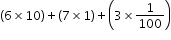.
MA.5.NSO.1.3: Compose and decompose multi-digit numbers with decimals to the thousandths in multiple ways using the values of the digits in each place. Demonstrate the compositions or decompositions using objects, drawings and expressions or equations.
 Examples:The number 20.107 can be expressed as 2 tens + 1 tenth+7 thousandths or as 20 ones + 107 thousandths.
MA.5.NSO.1.4: Plot, order and compare multi-digit numbers with decimals up to the thousandths.
 Clarifications:Clarification 1: When comparing numbers, instruction includes using an appropriately scaled number line and using place values of digits. Clarification 2: Scaled number lines must be provided and can be a representation of any range of numbers. Clarification 3: Within this benchmark, the expectation is to use symbols (<, > or =).

 Examples:Example: The numbers 4.891; 4.918 and 4.198 can be arranged in ascending order as 4.198; 4.891 and 4.918. Example: 0.15<0.2 because fifteen hundredths is less than twenty hundredths, which is the same as two tenths.
MA.5.NSO.1.5: Round multi-digit numbers with decimals to the thousandths to the nearest hundredth, tenth or whole number.
 Examples:The number 18.507 rounded to the nearest tenth is 18.5 and to the nearest hundredth is 18.51.
MA.5.NSO.2.1: Multiply multi-digit whole numbers including using a standard algorithm with procedural fluency.
MA.5.NSO.2.2: Divide multi-digit whole numbers, up to five digits by two digits, including using a standard algorithm with procedural fluency. Represent remainders as fractions.
 Clarifications:Clarification 1: Within this benchmark, the expectation is not to use simplest form for fractions.

 Examples:The quotient 27÷7 gives 3 with remainder 6 which can be expressed as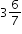.
MA.5.NSO.2.3: Add and subtract multi-digit numbers with decimals to the thousandths, including using a standard algorithm with procedural fluency.
MA.5.NSO.2.4: Explore the multiplication and division of multi-digit numbers with decimals to the hundredths using estimation, rounding and place value.
 Clarifications:Clarification 1: Estimating quotients builds the foundation for division using a standard algorithm.Clarification 2: Instruction includes the use of models based on place value and the properties of operations.

 Examples:The quotient of 23 and 0.42 can be estimated as a little bigger than 46 because 0.42 is less than one-half and 23 times 2 is 46.
MA.5.NSO.2.5: Multiply and divide a multi-digit number with decimals to the tenths by one-tenth and one-hundredth with procedural reliability.
 Clarifications:Clarification 1: Instruction focuses on the place value of the digit when multiplying or dividing.

 Examples:The number 12.3 divided by 0.01 can be thought of as ?×0.01=12.3 to determine the quotient is 1,230.
MA.K12.MTR.1.1: Actively participate in effortful learning both individually and collectively.

Mathematicians who participate in effortful learning both individually and with others:

• Analyze the problem in a way that makes sense given the task.
• Build perseverance by modifying methods as needed while solving a challenging task.
• Stay engaged and maintain a positive mindset when working to solve tasks.
• Help and support each other when attempting a new method or approach.

 Clarifications:Teachers who encourage students to participate actively in effortful learning both individually and with others: Cultivate a community of growth mindset learners.  Foster perseverance in students by choosing tasks that are challenging. Develop students’ ability to analyze and problem solve. Recognize students’ effort when solving challenging problems.
MA.K12.MTR.2.1: Demonstrate understanding by representing problems in multiple ways.

Mathematicians who demonstrate understanding by representing problems in multiple ways:

• Build understanding through modeling and using manipulatives.
• Represent solutions to problems in multiple ways using objects, drawings, tables, graphs and equations.
• Progress from modeling problems with objects and drawings to using algorithms and equations.
• Express connections between concepts and representations.
• Choose a representation based on the given context or purpose.
 Clarifications:Teachers who encourage students to demonstrate understanding by representing problems in multiple ways: Help students make connections between concepts and representations.Provide opportunities for students to use manipulatives when investigating concepts.Guide students from concrete to pictorial to abstract representations as understanding progresses.Show students that various representations can have different purposes and can be useful in different situations.
MA.K12.MTR.3.1: Complete tasks with mathematical fluency.

Mathematicians who complete tasks with mathematical fluency:

• Select efficient and appropriate methods for solving problems within the given context.
• Maintain flexibility and accuracy while performing procedures and mental calculations.
• Complete tasks accurately and with confidence.
• Adapt procedures to apply them to a new context.
• Use feedback to improve efficiency when performing calculations.
 Clarifications:Teachers who encourage students to complete tasks with mathematical fluency:Provide students with the flexibility to solve problems by selecting a procedure that allows them to solve efficiently and accurately.Offer multiple opportunities for students to practice efficient and generalizable methods.Provide opportunities for students to reflect on the method they used and determine if a more efficient method could have been used.
MA.K12.MTR.4.1: Engage in discussions that reflect on the mathematical thinking of self and others.

Mathematicians who engage in discussions that reflect on the mathematical thinking of self and others:

• Communicate mathematical ideas, vocabulary and methods effectively.
• Analyze the mathematical thinking of others.
• Compare the efficiency of a method to those expressed by others.
• Recognize errors and suggest how to correctly solve the task.
• Justify results by explaining methods and processes.
• Construct possible arguments based on evidence.
 Clarifications:Teachers who encourage students to engage in discussions that reflect on the mathematical thinking of self and others:Establish a culture in which students ask questions of the teacher and their peers, and error is an opportunity for learning.Create opportunities for students to discuss their thinking with peers.Select, sequence and present student work to advance and deepen understanding of correct and increasingly efficient methods.Develop students’ ability to justify methods and compare their responses to the responses of their peers.
MA.K12.MTR.5.1: Use patterns and structure to help understand and connect mathematical concepts.

Mathematicians who use patterns and structure to help understand and connect mathematical concepts:

• Focus on relevant details within a problem.
• Create plans and procedures to logically order events, steps or ideas to solve problems.
• Decompose a complex problem into manageable parts.
• Relate previously learned concepts to new concepts.
• Look for similarities among problems.
• Connect solutions of problems to more complicated large-scale situations.
 Clarifications:Teachers who encourage students to use patterns and structure to help understand and connect mathematical concepts:Help students recognize the patterns in the world around them and connect these patterns to mathematical concepts.Support students to develop generalizations based on the similarities found among problems.Provide opportunities for students to create plans and procedures to solve problems.Develop students’ ability to construct relationships between their current understanding and more sophisticated ways of thinking.
MA.K12.MTR.6.1: Assess the reasonableness of solutions.

Mathematicians who assess the reasonableness of solutions:

• Estimate to discover possible solutions.
• Use benchmark quantities to determine if a solution makes sense.
• Check calculations when solving problems.
• Verify possible solutions by explaining the methods used.
• Evaluate results based on the given context.
 Clarifications:Teachers who encourage students to assess the reasonableness of solutions:Have students estimate or predict solutions prior to solving.Prompt students to continually ask, “Does this solution make sense? How do you know?”Reinforce that students check their work as they progress within and after a task.Strengthen students’ ability to verify solutions through justifications.
MA.K12.MTR.7.1: Apply mathematics to real-world contexts.

Mathematicians who apply mathematics to real-world contexts:

• Connect mathematical concepts to everyday experiences.
• Use models and methods to understand, represent and solve problems.
• Perform investigations to gather data or determine if a method is appropriate. • Redesign models and methods to improve accuracy or efficiency.
 Clarifications:Teachers who encourage students to apply mathematics to real-world contexts:Provide opportunities for students to create models, both concrete and abstract, and perform investigations.Challenge students to question the accuracy of their models and methods.Support students as they validate conclusions by comparing them to the given situation.Indicate how various concepts can be applied to other disciplines.
ELA.K12.EE.1.1: Cite evidence to explain and justify reasoning.
 Clarifications:K-1 Students include textual evidence in their oral communication with guidance and support from adults. The evidence can consist of details from the text without naming the text. During 1st grade, students learn how to incorporate the evidence in their writing.2-3 Students include relevant textual evidence in their written and oral communication. Students should name the text when they refer to it. In 3rd grade, students should use a combination of direct and indirect citations.4-5 Students continue with previous skills and reference comments made by speakers and peers. Students cite texts that they’ve directly quoted, paraphrased, or used for information. When writing, students will use the form of citation dictated by the instructor or the style guide referenced by the instructor. 6-8 Students continue with previous skills and use a style guide to create a proper citation.9-12 Students continue with previous skills and should be aware of existing style guides and the ways in which they differ.
 Clarifications:See Text Complexity for grade-level complexity bands and a text complexity rubric.
ELA.K12.EE.3.1: Make inferences to support comprehension.
 Clarifications:Students will make inferences before the words infer or inference are introduced. Kindergarten students will answer questions like “Why is the girl smiling?” or make predictions about what will happen based on the title page. Students will use the terms and apply them in 2nd grade and beyond.
ELA.K12.EE.4.1: Use appropriate collaborative techniques and active listening skills when engaging in discussions in a variety of situations.
 Clarifications:In kindergarten, students learn to listen to one another respectfully.In grades 1-2, students build upon these skills by justifying what they are thinking. For example: “I think ________ because _______.” The collaborative conversations are becoming academic conversations.In grades 3-12, students engage in academic conversations discussing claims and justifying their reasoning, refining and applying skills. Students build on ideas, propel the conversation, and support claims and counterclaims with evidence.
ELA.K12.EE.5.1: Use the accepted rules governing a specific format to create quality work.
 Clarifications:Students will incorporate skills learned into work products to produce quality work. For students to incorporate these skills appropriately, they must receive instruction. A 3rd grade student creating a poster board display must have instruction in how to effectively present information to do quality work.
ELA.K12.EE.6.1: Use appropriate voice and tone when speaking or writing.
 Clarifications:In kindergarten and 1st grade, students learn the difference between formal and informal language. For example, the way we talk to our friends differs from the way we speak to adults. In 2nd grade and beyond, students practice appropriate social and academic language to discuss texts.
ELD.K12.ELL.MA.1: English language learners communicate information, ideas and concepts necessary for academic success in the content area of Mathematics.
ELD.K12.ELL.SI.1: English language learners communicate for social and instructional purposes within the school setting.

## General Course Information and Notes

### VERSION DESCRIPTION

The benchmarks in this course are mastery goals that students are expected to attain by the end of the year. To build mastery, students will continue to review and apply earlier grade-level benchmarks and expectations.

### GENERAL NOTES

In grade 5, instructional time will emphasize five areas: (1) multiplying and dividing multi-digit whole numbers, including using a standard algorithm; (2) adding and subtracting fractions and decimals with procedural fluency, developing an understanding of multiplication and division of fractions and decimals; (3) developing an understanding of the coordinate plane and plotting pairs of numbers in the first quadrant; (4) extending geometric reasoning to include volume and (5) extending understanding of data to include the mean.

Curricular content for all subjects must integrate critical-thinking, problem-solving, and workforce-literacy skills; communication, reading, and writing skills; mathematics skills; collaboration skills; contextual and applied-learning skills; technology-literacy skills; information and media-literacy skills; and civic-engagement skills.

English Language Development ELD Standards Special Notes Section:
Teachers are required to provide listening, speaking, reading and writing instruction that allows English language learners (ELL) to communicate information, ideas and concepts for academic success in the content area of Mathematics. For the given level of English language proficiency and with visual, graphic, or interactive support, students will interact with grade level words, expressions, sentences and discourse to process or produce language necessary for academic success. The ELD standard should specify a relevant content area concept or topic of study chosen by curriculum developers and teachers which maximizes an ELL’s need for communication and social skills. To access an ELL supporting document which delineates performance definitions and descriptors, please click on the following link:

### General Information

 Course Number: 5012070 Course Path: Section: Grades PreK to 12 Education Courses > Grade Group: Grades PreK to 5 Education Courses > Subject: Mathematics > SubSubject: General Mathematics > Abbreviated Title: GRADE FIVE MATH Course Attributes: Florida Standards Course Course Type: Core Academic Course Course Level: 2 Course Status: State Board Approved Grade Level(s): K,1,2,3,4,5

#### Educator Certifications

 enVision Florida B.E.S.T. Mathematics Grade 5R. Charles, et al - Savvas Learning Company LLC - 1 - 2023Florida Reveal Math, Grade 5Linda Gojak, M.Ed.; Annie Fetter, B.A.; Susie Katt, Ed.D.; Georgina Rivera, M.Ed.; John SanGiovanni, M.Ed.; Raj Shah, Ph.D.; Nicki Newton, Ed.D.; Cheryl Tobey M.Ed.; Ralph Connelly, Ph.D.; Ruth Harbin Miles, Ed.S.; Jeff Shih, Ph.D.; Dinah Zike, M.Ed.; Sharon Griffin, Ph.D. - McGraw Hill LLC - 1 - 2023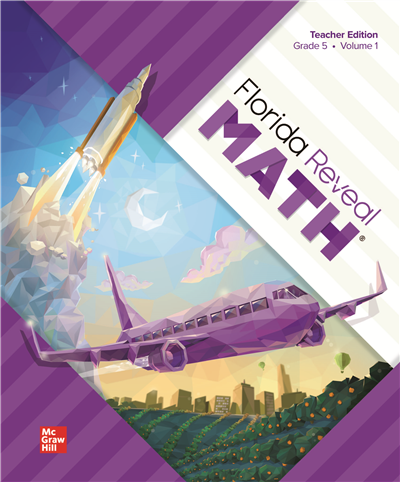Florida's B.E.S.T. Standards for MATH Grade 5Ron Larson and Laurie Boswell - Big Ideas Learning, LLC - 1 - 2023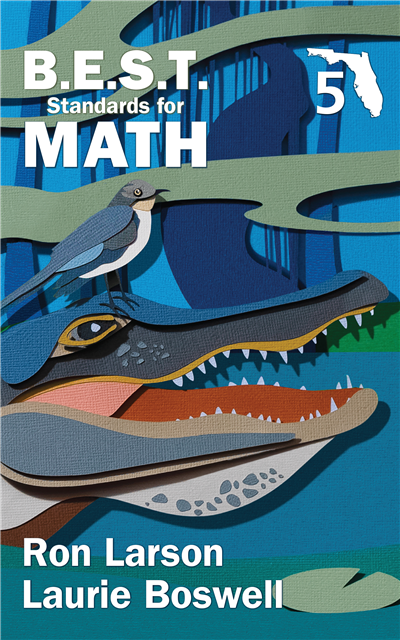HMH Florida's B.E.S.T. Go Math!Edward B. Burger, PhD; Juli K. Dixon, PhD; Thomasenia Lott Adams, PhD; Matthew R. Larson, PhD - Houghton Mifflin Harcourt - N/A - 2023STEMscopes Florida MathDr. Jarrett Reid Whitaker - Accelerate Learning - First Edition - 2022